# Fast Summation in Excel

In this article, we will learn how we can apply the fast summation to the numbers. For doing this, we will use the SUM function in Microsoft Excel.

SUM: This function is used to find the sum of the numbers in a range of cells.

The syntax of SUM formula:- =SUM(number1,number2,……)

To sum up the columns within the Current Region:

Let’s take an example to understand how we can add the numbers for rows.

We have sales data in range A2:A20. Now, we want to return the total value.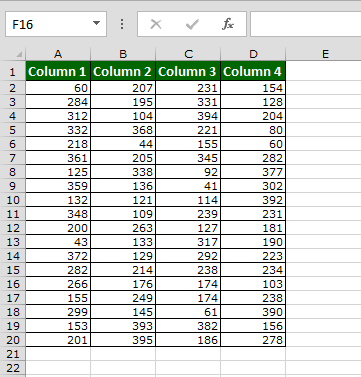• Select a cell in the Current Region/List and press Ctrl+Shift+*> or Ctrl+A.
• Press Alt+=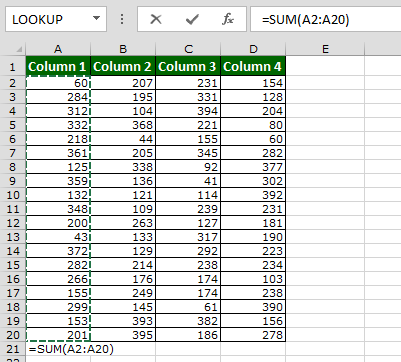• Select Formulas -> AutoSum (in Function Library Group)
• The SUM formula is added automatically at the bottom of each column.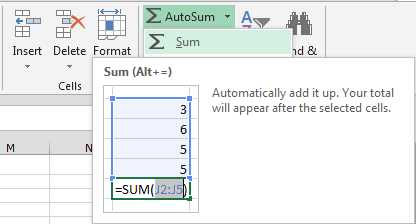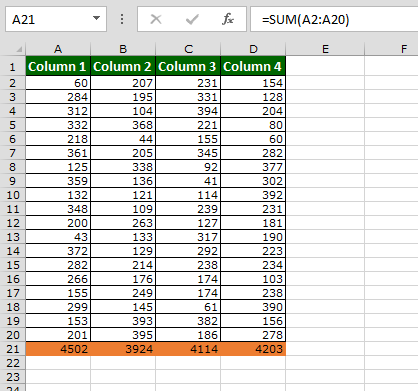To SUM all values in a list, in vertical and horizontal directions:

Let’s take an example to understand how we can add the numbers for rows.

• Select a range of cells containing values, including a blank row and a blank column surrounding the range by selecting the first cell in a table by pressing the Shift> key.
• Then select the blank cell in the first cross blank row and column
• Press Alt+= Or Select Formulas -> AutoSum (in Function Library Group).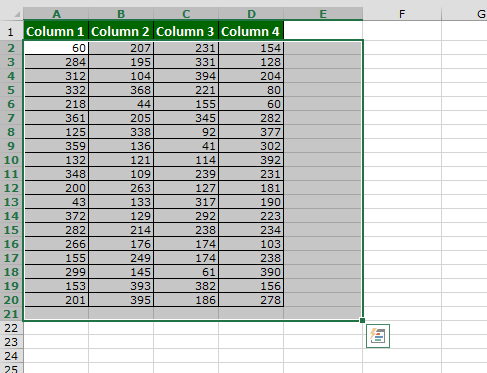• The SUM formula is added at the end of each row and at the bottom of each column.This is the way we can do fast summation in Microsoft Excel.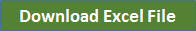Terms and Conditions of use

The applications/code on this site are distributed as is and without warranties or liability. In no event shall the owner of the copyrights, or the authors of the applications/code be liable for any loss of profit, any problems or any damage resulting from the use or evaluation of the applications/code.